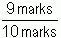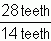SEARCH HOMEMath Central Quandaries & QueriesQuestion from nancy, a student: term=a fraction comprised as 2 numbers having the same unitsWE have two responses for you

Hi Nancy.

There are several terms we could use for such a fraction.

If I get 9 marks out of a possible 10 marks on a quiz, that is the fractionand I say I earned 90% on the quiz. So perhaps "percentage" is the term you are looking for.

Another possibility is "ratio". If my bicycle has a gear with 28 teeth in the front and 14 teeth in the rear, then the gear ratio is 28 teeth : 14 teeth or as a fraction this iswhich often gets written just as a decimal (this reduces to 2.0). This means that for one rotation of the pedals, the wheel will rotate twice, so higher gear ratios are harder, but faster.

There are other possible terms that fit what you describe as well. For example, the "scale" on a map or model is often represented as a fraction like this.

Cheers,
Stephen La Rocque.

Hi Nancy,

If you form a fraction where the numerator and denominator have the same units then the resulting fraction has no units so I wonder if the term you want is unitless.

HarleyMath Central is supported by the University of Regina and The Pacific Institute for the Mathematical Sciences.